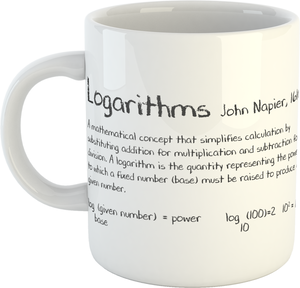# Logarithms Mug

Regular price £7.49 £0.00

Logarithms - John Napier, 1610

A mathematical concept that simplifies calculation by substituting addition for multiplication and subtraction for division. A logarithm is the quantity representing the power to which a fixed number (base) must be raised to produce a given number.

The perfect gift for anyone interested in the fields of Mathematics and Science.